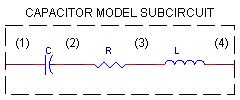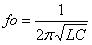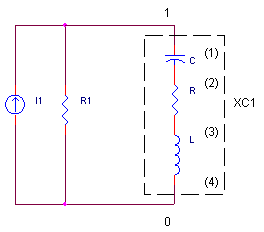eCircuit  Center

### Capacitor Model

CIRCUITIdeal capacitors exist only in textbooks, not on real circuit boards. You may be surprised to find that all practical capacitors look (behave) similarly to the series RLC network shown above. Whether you're designing a filter or picking bypass capacitors, you'll need to know what your capacitor looks like across the entire frequency range. As you can imagine, having inductance in your filter at high frequencies where your intent was a capacitor, can produce some unexpected results.

REAL CAPACITORS

The reality of producing capacitors creates two parasitic components that come along with the package: inductance and resistance. External leads and internal paths create inductive and resistive parasitic components. So how does the real capacitor behave versus frequency? You can break down the behavior into three frequencies.

frequency                    impedance
Low                              Capacitive
Self-Resonance             Resistive
High                              Inductive

At low frequencies, the capacitor's impedance looks just like you'd expect from the specified capacitor value. At self-resonance, the capacitive and inductive impedances cancel each other out leaving only a resistive component. The self resonance is given byThe resistive component is usually referred to as the Equivalent Series Resistor (ESR). Above self-resonance, the inductive reactance takes over as it grows much larger than the capacitive reactance and ESR.

CAPACITOR SPICE MODEL

What values of C, L and R do you choose to create the capacitor model? The table below shows you how to assign values.

 component value C Capacitance R ESR L L = 1/ [( 2π fo)² x C]where fo is the self-resonant frequency

The capacitance value comes right from its specified value. The ESR and self-resonant frequency fo are usually available from the manufacturer's web site or by request.

Let's create capacitor models for 1 uF and 0.1 uF capacitors. For a 1 uF capacitor, ESR = 0.03 ohms, fo = 1 MHz and we calculate L = 25.3 nH. Similarly, for the 0.1 uF capacitor, ESR = 0.08 ohms, fo = 10 MHz and we calculate L = 2.53 nH.

It's convenient to create a subcircuit for the three elements of the capacitor. Why? This makes it easy to insert the model into a new or existing circuit. (See Why Use Subcircuits?) For the 1 uF capacitor, the subcircuit call and definition are shown below.

XC1 1 0 C105            ;subcircuit inserted at nodes 1 and 0

.SUBCKT C105 1 4         ;subcircuit definition
* FO = 1 MHZ
C 1 2 1UF
R 2 3 0.03OHMS
L 3 4 25.3NH
.ENDS

CAPACITOR IMPEDANCE VS FREQUENCY

How do you plot the impedance of a network? One trick is to drive the network with a 1A RMS current source and then plot the voltage across the network. This works because the voltage is equal to the impedance under these conditions ( V = Z x I = Z x 1 = Z).CIRCUIT INSIGHT   Run a simulation of the SPICE file CMODEL1.CIR. Look at the the impedance of the 1 uF model (C105) by plotting VM(1). Change the Y-axis to a log scale to get a better view. If this were an ideal capacitor, what would you see? The impedance would get smaller as frequency increases Xc = 1/(2π x C x f). However, the impedance of this model takes a dip at self-resonance and then starts increasing with frequency! The inductive impedance takes over (Xl = 2π x L x f ) . This may produce disappointing results if this capacitor is chosen for a filter operating at frequencies near or above its self-resonance.

How do we know the impedance is capacitive and inductive at frequencies below and above self-resonance? Plot the phase shift of the network by adding a new plot window and adding trace VP(1). Negative and positive phase indicate capacitive and inductive reactances, respectively.

IMPEDANCE VS. CAPACITOR SIZE

CIRCUIT INSIGHT   For a given capacitor type, what happens to the self-resonant frequency for smaller capacitor values? Plot the impedance of a 0.1uF capacitor (C104) by adding trace VM(2). The self-resonance is higher making this capacitor more useful at higher frequencies. To see these models in action, check out Power Supply Bypassing.

CAPACITOR NOTES

These subcircuits model a capacitor's self-resonant and series resistive behavior. More complex models can be created that mimic other non-ideal behaviors such as dielectric absorption, leakage and temperature effects. Some capacitor manufacturers provide SPICE models that include these effects.

SPICE MODEL CALCULATOR

Check out this handy webpage that creates a capacitor SPICE model. Simply enter the essential parameters and the JavaScript/HTML code spits out a subcircuit for you to copy into your netlist or SPICE library file.

SIMULATION NOTE

You may have noticed R1 = 100MEG to ground across the capacitor model. What's the purpose of this component if it has little effect on the impedance? The problem is that without R1, node 1 has no resistive path to ground; SPICE is not happy under these conditions and may grind to a halt. The 100MEG resistor solves the problem by providing a DC path to ground.

SPICE FILE

Download the file or copy this netlist into a text file with the *.cir extention.

```CMODEL.CIR - CAPACITOR MODEL
*
* MEASURE IMPEDANCE OF CAPACITORS USING 1A CURRENT SOURCES
I1	0	1	AC	1
XC1	1	0	C105
R1	1	0	100MEG
*
I2	0	2	AC	1
XC2	2	0	C104
R2	2	0	100MEG
*
* 1 UF CAPACITOR MODEL - INCLUDES ESR AND SELF-RESONANCE
.SUBCKT C105	    1   4
* FO = 1 MEG HZ
C	1	2	1UF
R	2	3	0.03OHMS
L	3	4	25.3NH
.ENDS
*
* 0.1 UF CAPACITOR MODEL - INCLUDES ESR AND SELF-RESONANCE
.SUBCKT C104	    1   4
* FO = 10 MHZ
C	1	2	0.1UF
R	2	3	0.08OHMS
L	3	4	2.53NH
.ENDS
*
* ANALYSIS
.AC 	DEC 40	10K 100MEG
* VIEW RESULTS
.PRINT AC	VM(1) VP(1)
.PLOT	AC 	VM(1) VP(1)
.PROBE
.END```

top